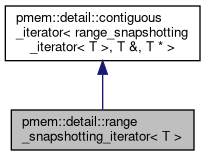PMDK C++ bindings  1.13.0-git85.g2ab46040 This is the C++ bindings documentation for PMDK's libpmemobj.
pmem::detail::range_snapshotting_iterator< T > Struct Template Reference

Non-const iterator which adds elements to a transaction in a bulk. More...

#include <libpmemobj++/container/detail/contiguous_iterator.hpp>

Inheritance diagram for pmem::detail::range_snapshotting_iterator< T >:[legend]

Public Types

using iterator_category = std::random_access_iterator_tag

using value_type = T

using difference_type = std::ptrdiff_t

using reference = T &

using pointer = T *

using base_type = contiguous_iterator< range_snapshotting_iterator< T >, reference, pointer >

Public Member Functions

range_snapshotting_iterator (pointer ptr=nullptr, pointer data=nullptr, std::size_t size=0, std::size_t snapshot_size=1)
Constructor taking pointer to data, pointer to the beginning of the array and snapshot_size.

operator const T * () const
Conversion operator to const T*.

reference operator[] (std::ptrdiff_t n)
Element access operator. More...

T & operator* () const
Dereference operator.

T * operator-> () const
Arrow operator.

range_snapshotting_iterator< T > & operator++ ()
Prefix increment operator.

range_snapshotting_iterator< T > operator++ (int)
Postfix increment operator.

range_snapshotting_iterator< T > & operator-- ()
Prefix decrement operator.

range_snapshotting_iterator< T > operator-- (int)
Postfix decrement operator.

range_snapshotting_iterator< T > & operator+= (std::ptrdiff_t n)

range_snapshotting_iterator< T > & operator-= (std::ptrdiff_t n)
Subtraction assignment operator.

range_snapshotting_iterator< T > operator+ (std::ptrdiff_t n) const

range_snapshotting_iterator< T > operator- (std::ptrdiff_t n) const
Subtraction operator overload for integral type.

T * get_ptr () const

Protected Member Functions

void change_by (std::ptrdiff_t n)

T * ptr

Friends

template<typename Iterator , typename Reference , typename Pointer >
struct contiguous_iterator

void swap (range_snapshotting_iterator &lhs, range_snapshotting_iterator &rhs)
Non-member swap function.

Detailed Description

template<typename T> struct pmem::detail::range_snapshotting_iterator< T >

Non-const iterator which adds elements to a transaction in a bulk.

This is done by dividing underlying array into ranges of specified (snapshot_size) size. If iterator is incremented/decremented/etc. so that it is moved to another range, this new range is added to a transaction.

For example, let's assume snapshot_size = 2, N = 6. This gives us: 0 1 | 2 3 | 4 5

If iterator is moved from 1 to 3, that means it is now in another range, and that range must be added to a transaction (elements 2 and 3).

◆ operator[]()

template<typename T >
 reference pmem::detail::range_snapshotting_iterator< T >::operator[] ( std::ptrdiff_t n )
inline

Element access operator.